Practice Exam I Math002

Q1. Given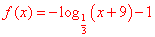.

a)      Find x-intercept and y-intercept.

b)      Graph f(x).

c)      Find the domain and the range of  f.

d)      Find an equation of the asymptote of the graph of f.

Q2. If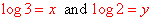, then write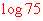in terms of  x and y.

Q3. Find the solution set of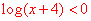.

Q4. Solve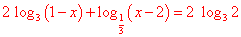Q5. Solve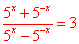Q6.   a)  Find the measure of the complement of the angle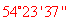.

b)  Find the length of an arc that subtends a central angle of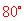in a circle

Q7. A car with a wheel of radius 13 inches is moving with a speed of 50 mph. Find the angular speed of the wheel in radian per minute.

Q8.  Find the exact value of  each expression

a)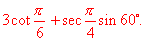b)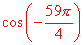Q9. A 8 – foot ladder is resting against a wall and makes an angle of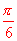with the ground. Find the height to which the ladder will reach on the wall.

Q9.    a) Is the function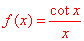even, odd, or neither?

b) Write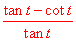in terms of a single trigonometric function.

c) If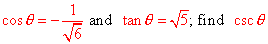.

Q10. Given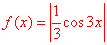.

a)      Graph f.

b)      Find period and amplitude of the graph of f.

c)       Find the range of f.